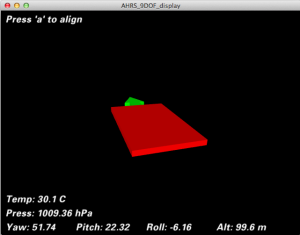## 陀螺儀(gyro)

```int offset = 0;
int noise = 0;
int angle = 0;
int rate = 0;
int sampleNum = 200;
void setup() {
for(int n = 0; n < sampleNum; n++){
offset += (int) gyro.g.x;
}
offset /= sampleNum;
int val = 0;
for(int n = 0; n < sampleNum; n++){
val = gyro.g.x - offset;
if(val - offset > noise)
noise = val - offset;
else if(val - offset[i] < -noise[i])
noise = -val - offset;
}
noise /= 100;
}
void loop() {
int prev_rate = 0
int rate;
rate = (gyro.g.x - offset) / 100;
if(rate >= noise || rate <= -noise)
angle += ((double)(prev_rate + rate) * 10) / 2000;
prev_rate = rate;
if(angle >= 360)
angle -= 360;
else if(angle < 0)
angle += 360;
}
```

## IMU – GY80

https://github.com/ptrbrtz/razor-9dof-ahrs
razor 9dof 是一個 IMU（整合的感測器）的函式庫，他支援幾種 IMU 並且有多種平台的實作可以參考。

#YPR=15.25,1.02,-5.77

https://github.com/vshymanskyy/GY80

82.64;0.54;-6.00;29.90;1009.36;98.84https://github.com/philtkp/GY80-arduino

## 紀錄問題：

• 感測器怎麼接？：大都是三條線，一條接 5v 一條接地 一條選一個 pin 腳當信號輸入
• gy80好多腳要接幾隻腳：一電源(VCC_IN)一接地(GND) 兩個信號 (SDA, SCL) 接到 A4 A5 就夠讀到所有感測器的值了
• 電調怎麼接：三條粗的線隨便跟馬達三個腳對接，剩下兩個腳接電源，三條細線一樣 電 接地 信號
• 怎麼讓馬達轉：送脈波訊號，或者 servo.h write 到 pin 腳
• 機架怎麼裝：底板有一個很多洞的可以把電調焊上去 有正負兩個迴路，再把電源線焊上去 + – 兩個洞
• 電池？：三顆3.7v 串連起來接到底板上，可以提供電調（馬達）電，也能供應給 arduino 電

## 零散資源

pid
http://oaione.blogspot.tw/2014/02/multiwii-vi-pid.html
GY80
http://dutch-arduino-tinkering.blogspot.tw/2014/01/gy-80-bmp085-9-axis-magnetic.html
A Guide To using IMU (Accelerometer and Gyroscope Devices) in Embedded Applications.
http://www.starlino.com/imu_guide.html

HW30A 測試 code: https://www.sendspace.com/file/jrtfu0

HW 30a datasheet